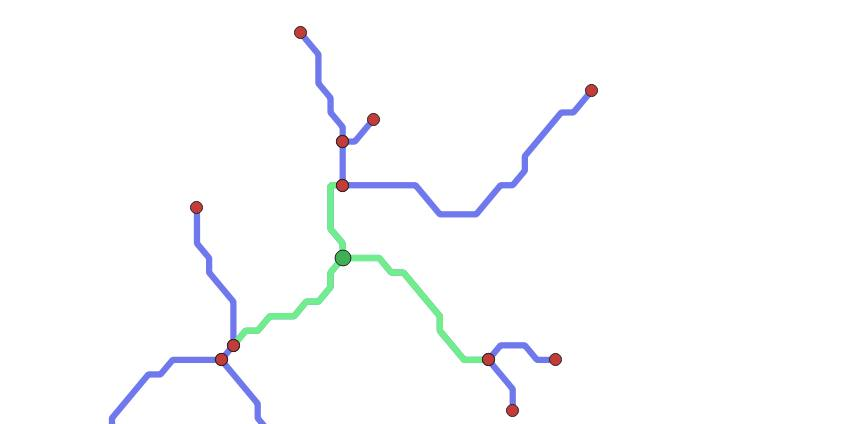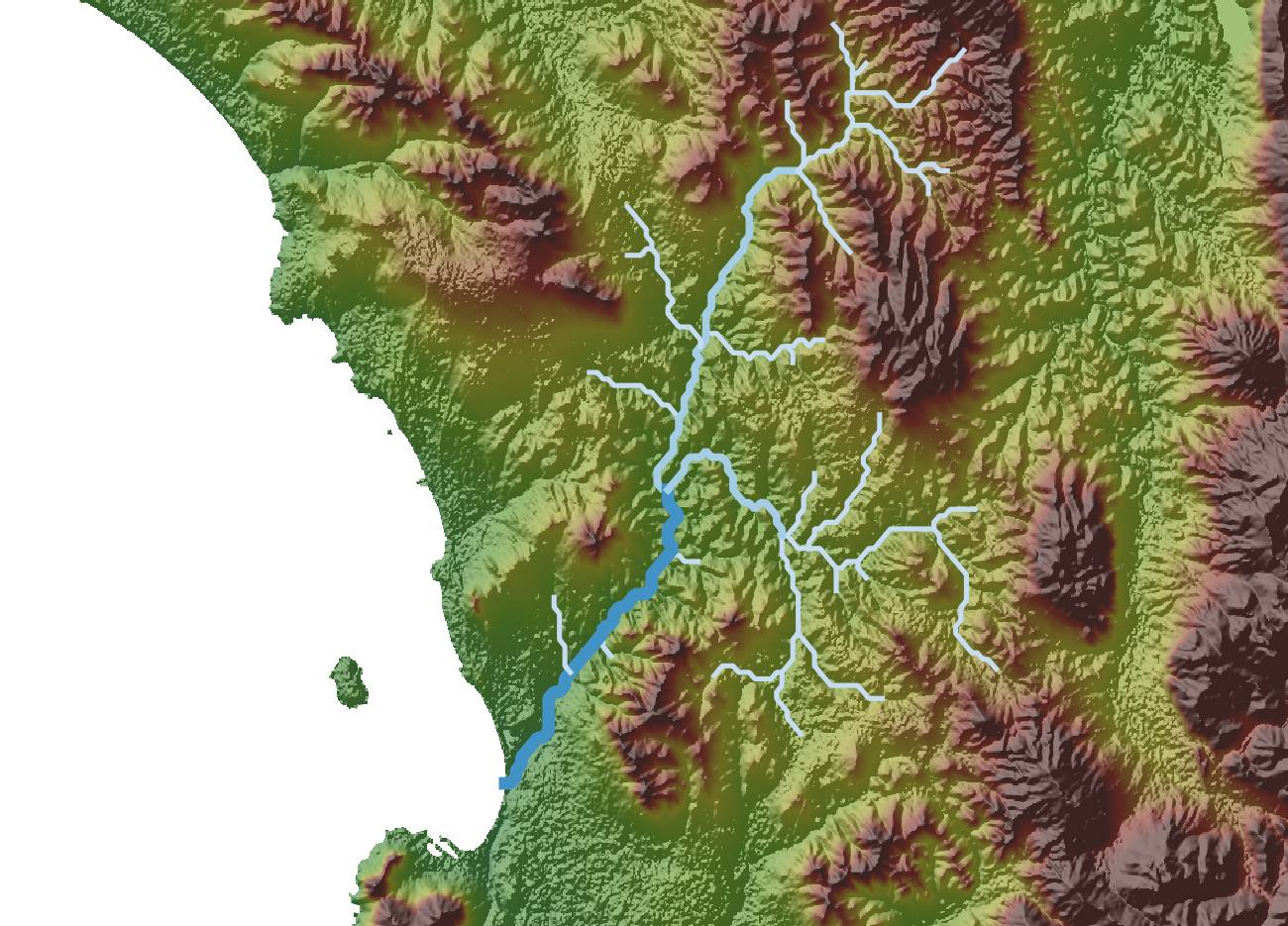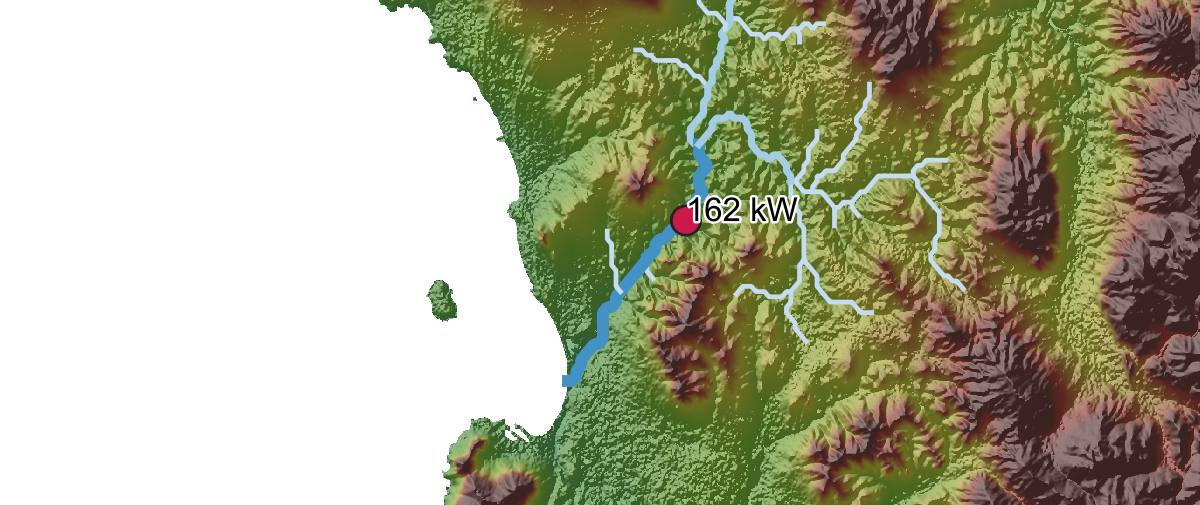Modelling hydrological networks at massive scale

Ironically, a large part of my work at KTH’s Division of Energy Systems Analysis was modelling complex hydrological systems. Often, this was while modelling the ‘nexus’ between different systems, such as energy, water and climate – as opposed to treating these as separate fields of study.

A lot of this was done in WEAP, a hydrological modelling package courtesy of the Stockholm Environmental Institute. This provided an easy user interface, and access to a variety of complex models and systems for things such as rainwater run-off and crop requirements. However, it isn’t easily scriptable, and is mostly suited to very localised systems.

So when my colleagues and I wanted to conduct a continent-scale assessment of mini-hydropower potential, we had to turn elsewhere. For the first version of this, I created a simple script to run down every river in a given network and calculate the elevation drop (head) between points to estimate hydropower potential. We applied this to the whole of Sub-Saharan Africa, using river networks from HydroSHEDS and run-off data from the EU’s Joint Research Centre.

Update: The paper we wrote on this has recently been published with the journal Energies: A Geospatial Assessment of Small-Scale Hydropower Potential in Sub-Saharan Africa.

Later on, I decided to improve and generalise this simple model and came with an unwieldy bit of code called hydro-networks. Instead of just creating points all over the show, I set out to create a model that would:

• Conduct massive scale hydrological modelling
• Calculate run-off and stream-flow using precipitation and land-use data
• Model rivers in a network-aware manner
• Allow point sources/drains such as farms and dams to be added procedurally

At a rough estimate, I achieved about 3/4 of this, and realised run-off modelling is damn hard. What I did manage was still pretty fun, so I’m going to go over an example below, in the hopes that one day I’ll pick this up again and complete it.

## Create direction-aware river networks

The model uses the wonderful GeoPandas library to read in the river network data from HydroSHEDS. It is very happy crunching the data for the whole African continent, but to make this easier, I’ve clipped out a small river called Dieprivier just outside Cape Town(yes, they exist).

The model then goes through a couple of steps to convert this GIS data into something we can use for modelling.

1. Go through every river segment in the input data, and create for it a separate arc, which altogether form the network data structure.
2. As it does this, create another data structure called nodes that contains all of these points where arcs meet or terminate.
3. Then tell every arc which two nodes it is connected to, and tell each node which arcs it is connected to. This means if we know a node location, we know which arcs connect to it, and therefore which other nodes are connected, and so on.

A small section of the resulting layers is shown below, with the nodes at the intersections of river arcs. The large green node knows which arcs are connected to it (also in green) and they in turn know about it.Nodes and arcs (edges) for a small section of the river.

## Giving the network some order

This is all very well, but it’s still just a bunch of lines and dots. To make this network a lot easier to use, we must assign stream order to the arcs – essentially indicating how many upstream branches they have. This is a useful proxy for how ‘big’ any part of a river is relative to the network, but also allows the model to easily orientate itself to what is up- and down-stream of any node.

There are two main approaches to this, the Strahler number and the Shreve number. For the Strahler number, the smallest tributaries are assigned an order of 1. These are then followed down, and when two 1’s join, they form a 2. If two streams with a different number join, it gets the higher of the two, i.e. 1+2=2. The Shreve number is similar, except the numbers always add, so 1+2=3! For this model, I used the Shreve number, as it ensures that a downstream section always has a higher number that the sections upstream of it.

To achieve this, I adapted the algorithm from this paper (Gleyzer, et al., 2007), a beautiful but not very intuitive recursive function. Basically, it starts at the mouth of a given river (identified by a separate bit of code) and then follows recursively up the network until it reaches the mountain-top tributaries. Then it trickles back down to the mouth, adding up the stream orders as it goes.

``````def shreve(arc_index, direction_node_id, network, nodes):
up_stream_orders = []
if len(nodes[direction_node_id]) == 5:
network[arc_index] = 1
else:
for index, arc in enumerate(nodes[direction_node_id]):
if index >= 4:
if network[arc] != arc_index:
if network[arc] != direction_node_id:
up_stream_orders.append(shreve(arc,
network[arc], network, nodes))
else:
up_stream_orders.append(shreve(arc,
network[arc], network, nodes))
max_orders = heapq.nlargest(2, up_stream_orders)
if len(max_orders) == 2:
order = 0 + max_orders + max_orders
else:
order = 0 + max(up_stream_orders)

network[arc_index] = order
return network[arc_index]
``````

Applied to the entire river network, it looks something like this.

## Adding data layers and doing things

Now that we have the network set up and ordered, it’s time to do something with it! In addition to the run-off data mentioned further up, there are a few other important layers:

• Digital elevation model (DEM), normally from NASA’s SRTM
• Flow accumulation, which is derived from the DEM and also available at HydroSHEDS
• Land cover, used for modelling run-off and available from the FAO
• ETo, the reference evapo-transpiration for an area, also available from the FAO
• Precipitation, from many sources, hopefully monthly, never easy to useFor example, here's our same river overlaid with the DEM elevation data.

Previously I used the laborious and buggy ArcPy t oextract all of this information in the network and nodes data structures, but now rasterio makes this a walk in the park. It’s a straightforward library for reading raster GIS data and sampling and manipulating it, and takes only a few lines to load all of this new data.

## Calculating hydropower potential

One thing that we can do with all of this data is replicate the hydropower estimates, from the paper we wrote, except now just for Dieprivier in Cape Town.Note that this is exclusively for run-of-river mini-hydropower. To estimate power output, we use the hydropower formula as follows:

• P = ηρgQH

where P is the power output, η is the efficiency, ρ is the density of water,and g is acceleration due to gravity. Q is the flow-rate, and H is the head, or height difference available. These last two must be calculated for each point using the data described above.

The head is simple: we get the height for our chosen point, and then look a set distance up-stream and get the height there – the difference between these is the head. The flow-rate is more complicated. The global run-off data we have is provided in m/s, where we need m3/s. So we do the following:

• Q = runoff × catchment-area

where catchment-area is the total area upstream of the selected point.Multiplying these together calculates the theoretical amount of water that should flow past this point.

Applying this to Dieprivier, the model creates a point every 500 metres and calculates the head over the preceding 500 metres. By filtering to only include those with at least 10 metres of head and more than 100 kW output, we get the following suggested point of 162 kW, with 12 metres of head and flow rate of 2.75 m3/s.Note that this is an unchecked, un-calibrated example result, I'm not at all suggesting someone should build a mini-hydropower site there!

## Going further with rainfall run-off and discharge

The result above was calculated using the GSCD, which provides only a single value, with no monthly or yearly variations. This doesn’t provide much information about seasonal changes, nor allow for any calibrations with stream gauges, which will likely be on a daily or monthly basis.

To improve this, I extended the model to work with precipitation as an input instead, which is often available as monthly (or even daily data). For each node then, the model takes local precipitation, land cover and evapo-transpiration data and uses the Simplified Coefficeint Method to calculate local rainfall run-off. This is then carried downstream in the model, and when stream join,their flows are combined. At the same time, there are certain losses on each stream due to various causes.

Then every river section has a (potentially) more accurate discharge associated with it, which is now able to vary by month and day. So in the example above, we could specify not only the mean power output, but also show which months we expect to have lower and higher output.

The code for this is all with the model in the GitHub rep, but be aware – it takes a lot of fiddling and calibration to get useful numbers out, and precipitation and stream-gauge data often require a lot more data wrangling to make ready for the model.I was on the verge of setting up the model to calibrate against some measurements automatically, but that’ll have to be a project for another day.

## Towards a generalised model

From there, it’s not a huge step to add other point withdrawals (such as city water requirements or a farm) and use the model to make predictions about the system. For example, by including projected precipitation changes and increased population (and hence increased withdrawals) we could model whether there would be a water shortfall, and in which months this would be most severe.

As I said, the model isn’t quite there, but hopefully I’ll come back to it at some point.